# GED Math : Statistics

## Example Questions

### Example Question #15 : Mean

Determine the mean of the numbers: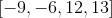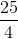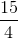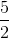Explanation:

The mean is the average of all the numbers in the data set.

Sum all the numbers and divide the quantity by 4.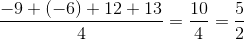The answer is:### Example Question #16 : Mean

There are nine people in an elevator.  They have an average weight of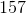lbs.  Two more people enter the elevator.  They have an average weight of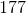lbs.  What is the new average weight of the group in the elevator?  Round to the nearest hundredth of a pound.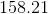lbs.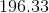lbs.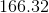lbs.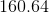lbs.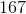lbs.lbs.

Explanation:

To figure out this problem, you must first calculate the total pounds in the elevator.

The first group is: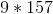or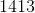lbs.

The second group is: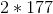or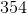lbs.

Thus, the total amount is: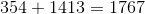Thus, the average weight in the elevator will be: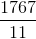(Remember, there are nowpeople in the elevator.)

This is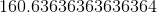orlbs.

### Example Question #17 : Mean

Determine the mean: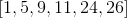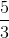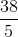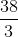Explanation:

Sum all the numbers and divide the quantity by 6.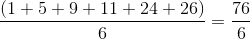Reduce this fraction.

The answer is:### Example Question #18 : Mean

Evaluate the mean: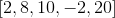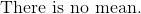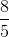Explanation:

The mean is the average of all the numbers in the data set.

Add all numbers and divide the total by five.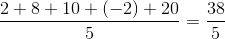The answer is:### Example Question #19 : Mean

Find the mean of the numbers: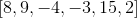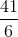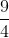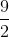Explanation:

The mean is the average of all the numbers.

Add all the numbers and divide the sum by 6.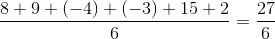Reduce this fraction.

The answer is:### Example Question #11 : Mean

The grade a student earns for a course depends on the mean of the best four of the five tests he takes. The minimum mean score for each grade is as follows:

A: 90

B: 80

C: 70

D: 60

Charles earned 68, 50, 77, 73, and 80 on his five tests. What was his letter grade?

F

C

D

B

C

Explanation:

Charlie's lowest score was a 50, so his grade was the mean of 68, 77, 73, and 80, which is the sum of the scores divided by the number of scores, 4;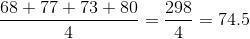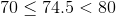,

### Example Question #21 : Mean

Determine the mean of the numbers: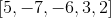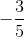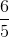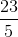Explanation:

The mean is the average of all the numbers given in the data set.

Add all the numbers and divide the quantity by five.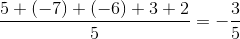The answer is:### Example Question #1891 : Ged Math

Use the following data set of student test scores to answer the question: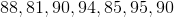Find the mean.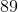Explanation:

To find the mean of a data set, we will use the following formula: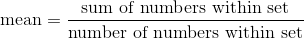Now, given the setwe can calculate the following: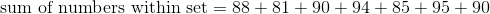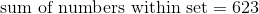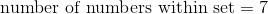because if we were to count the numbers in the set, we would get 7.

Now, we can substitute. We get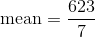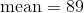Therefore, the mean of the data set is 89.

### Example Question #72 : Statistics

Find the mean of the numbers: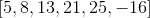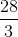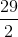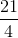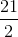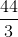Explanation:

The mean is the average of all the given numbers.

Add all the numbers and divide the sum by 6.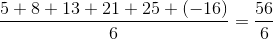Reduce the fraction.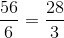The answer is:### Example Question #24 : Mean

Identify the mean: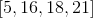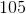Explanation:

The mean is the average of all the numbers given in the set of numbers.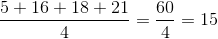The answer is:### All GED Math Resources